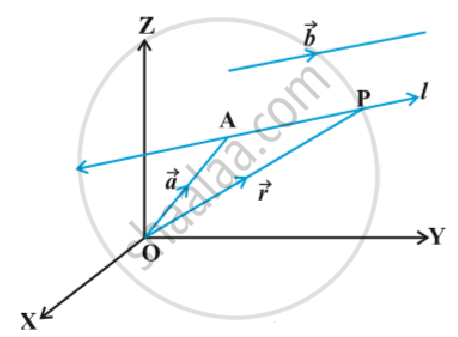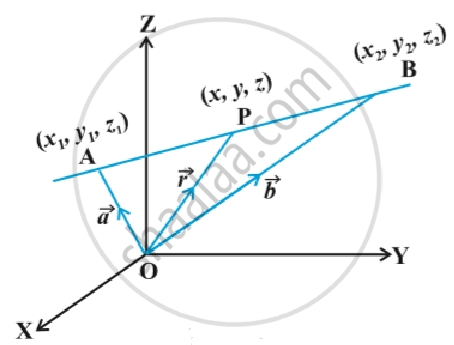# Equation of a Line in Space

• Equation of a line through a given point and parallel to a given vector vec b
• Equation of a line passing through two given points

#### notes

Equation of a line through a given point and parallel to a given vector vec b:
Let vec a be the position vector of the given point A with respect to the origin O of the rectangular coordinate system. Let l be the line which passes through the point A and is parallel to a given vector vec b .
Let  vec r be the position vector of an arbitrary point P on the line in following fig.Then vec (AP)  is parallel to the vector vec b , i.e., vec (AP) = λ vec b , where λ is some real number.
But  vec (AP) = vec (OP) - vec (OA)
i.e. lambda vec b =  vec r - vec a
Conversely, for each value of the parameter λ, this equation gives the position vector of a point P on the line.  Hence, the vector equation of the line is given by
vec r = vec a + lambda vec b    ...(1)

Remark:  If vec b = a hat i + b hat j + c hat k  , then a,b ,c are direction ratios of the line and conversely , if a, b , c are direction ratios of a line , then vec b = a hat i +b hat j +c hat k  will be the parallel to the line. Here, b should not be confused with | vec b|.

Derivation of cartesian form from vector form
Let the coordinates of the given point A be (x_1, y_1, z_1) and the direction  ratios of the line be a, b, c. Consider the coordinates of any point P be (x, y, z). Then
vec r = x hat i + y hat j + z hat k ;
vec a = x_1 hat i + y_1 hat j + z_1 hat k
and vec b = a hat i + b hat j + c hat k
Substituting these values in (1) and equating the coefficients of hat i , hat j " and " hat k , we get
x = x_1 + lambda a ; y = y_1 +lambda  b ; z = z_1 + lambda c  ...(2)
These are parametric equations of the line. Eliminating the parameter λ from (2), we get

(x - x_1)/a = (y - y_1)/a = (z - z_1)/c      ...(3)
This is the Cartesian equation of the line.

Equation of a line passing through two given points:
Let vec a and vec b be the position vectors of two points A(x_1 , y_1 , z_1) and B (x_2 , y_2 , z_2) respectively that are lying on a line in following fig.Let vec rbe the position vectors of an arbitrary point P(x , y , z), then P is a point on the  line if and only if vec (AP) = vec r - vec a and   vec (AB) = vec b - vec a are collinear vectors. Therefore, P is on the line if and only if vec r = vec a = lambda (vec b - vec a)
or vec r = vec a + lambda (vec b - vec a) , lambda ∈ R   ...(1)
This is the vector equation of the line.

Derivation of cartesian form from vector form
We have
vec r = x hat i + y hat j + z hat k , vec a = x_1 hat i +y_1 hat j + z_1 hat k and vec b = x_2 hat i + y_2 hat j + z_2 hat k,
Substituting these values in (1), we get
x hat i + y hat j+ z hat k = x_1hat i +y_1 hat j + z_1 hat k , + lambda [(x_2 - x_1) hat i +(y_2 - y_1) hat j + (z_2 -z_1 hat k)]
Equating the like coefficients of hat i , hat j , hat k ,we have
x = x_1 + lambda (x_2 - x_1); y = y_1 + lambda (y_2-y_1) ; z = z_1 +lambda (z_2 - z_1)
On eliminating  λ, we obtain

(x - x_1)/(x_2 - x_1) = (y - y_1)/ (y_2 - y_1) = (z - z_1) / (z_2 - z_1)

which is the equation of the line in Cartesian form.

If you would like to contribute notes or other learning material, please submit them using the button below.

### Shaalaa.com

Three Dimensional Geometry Part 2 -Straight Line [00:21:23]
S
0%